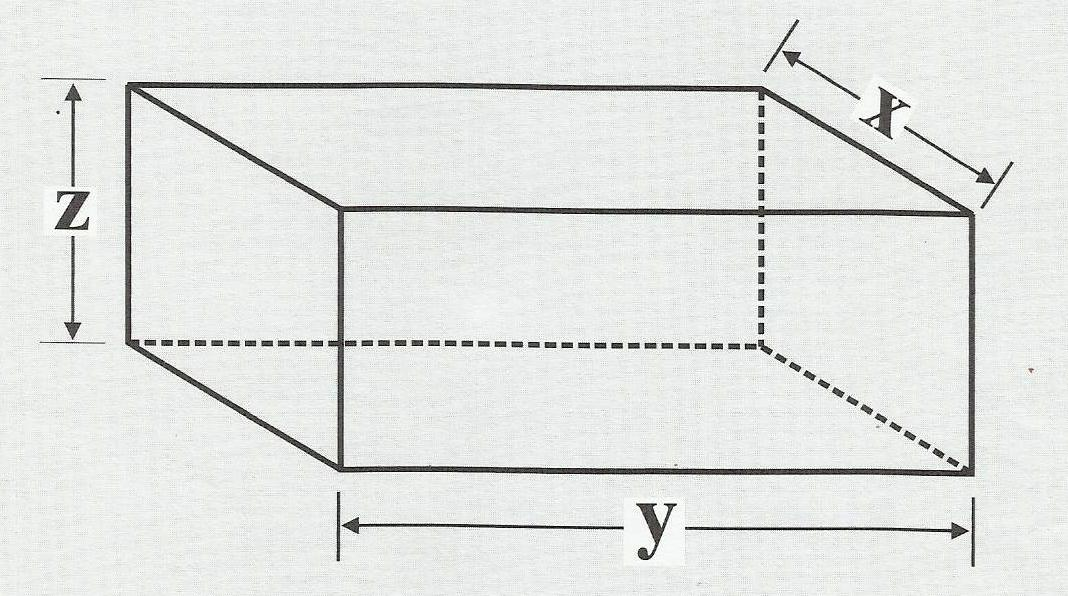# Prismatic PackageA prismatic package has side lengths $x,y,$ and $z$ and volume $108.$ Its surface area $S$ (without the cover) is given by $S = xy + 2yz + 2xz.$

What is the value of $x+y+z$ that minimizes $S?$

×